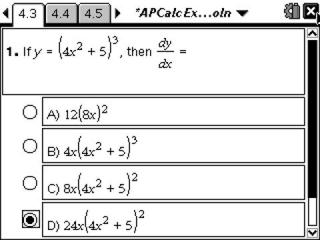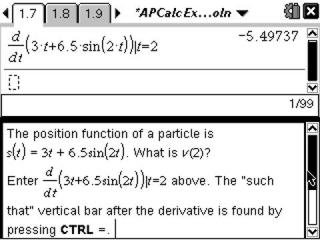Activities

••• Subject Area

• Math: Calculus: Transcendental Functions
• Math: Calculus: Applications of Integral, Antiderivatives and Definite Integrals
• Math: Calculus: Applications of the Derivative
• Math: Calculus: Derivatives
• Math: Calculus: Antiderivatives and Slope Fields
• Math: Calculus: Limits of Functions

• Author9-12

20 Minutes

• Device
•TI-Nspire™ CX/CX II
•TI-Nspire™ CX CAS/CX II CAS
• TI-Nspire™
• TI-Nspire™ CAS
• Software

TI-Nspire™
TI-Nspire™ CAS

2.1

• Report an Issue

AP Calculus Exam Prep

Activity Overview

Students prepare for the AP Calculus exam by reviewing and gaining skills in graphing, finding intersections, zeros, derivatives at a point, and evaluating definite integrals.

Key Steps

•This activity is written at an introductory level for new or less experience users of the TI-Nspire CAS handheld.

Students will do exercises and learn practice tips to help them prepare for the AP Calculus exam. They also will review and gain skills in graphing, finding intersections, zeros, derivatives at a point and evaluating definite integrals.

•In Part 2, examples of non-calculator self-check multiple-choice questions are provided.